# Bihar Board Class 10th Maths Solutions Chapter 6 Triangles Ex 6.3

Bihar Board Class 10th Maths Solutions Chapter 6 Triangles Ex 6.3 Textbook Questions and Answers.

## BSEB Bihar Board Class 10th Maths Solutions Chapter 6 Triangles Ex 6.3Question 1.
State which pairs of triangle in the figures, are similar. Write the similarity criterion used by you for answering the question and also write the pairs of similar triangles in the symbolic form :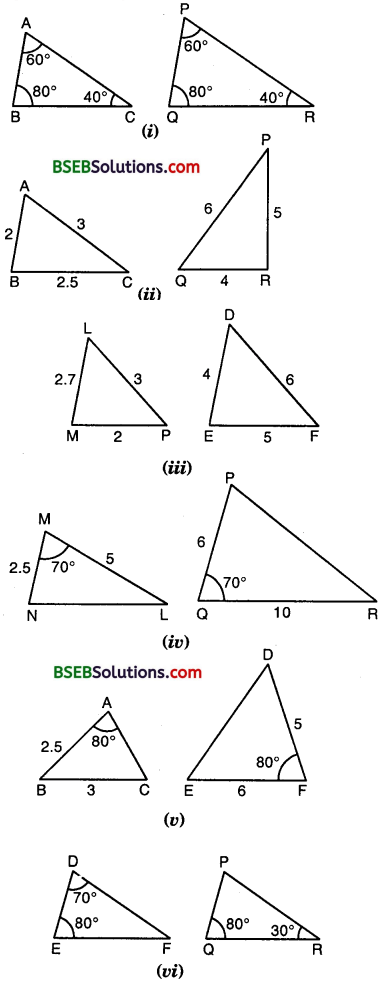Solution:
(i) In ∆s ABC and PQR, we observe that
∠A = ∠P = 60°, ∠B = ∠Q = 80° and ∠C = ∠R = 40°. By AAA criterion of similarity,
∆ ABC ~ ∆ PQR.

(ii) In ∆s ABC and PQR, we observe that
$$\frac { AB }{ QR }$$ = $$\frac { BC }{ RP }$$ = $$\frac { CA }{ PQ }$$ = $$\frac { 1 }{ 2 }$$
∴By SSS criterion of similarity, ∆ ABC ~ ∆ QRP.

(iii) In ∆s LMP and DEF, we observe that the ratio of the sides of these triangles are not equal.
[ $$\frac { MP }{ DE }$$ = $$\frac { LP }{ DF }$$ but it is not equal to $$\frac { LM }{ EF }$$ ]
So, the two triangles are not similar.

(iv) In ∆s MNL and QPR, we observe that
∠M = ∠Q = 70°
But, $$\frac { MN }{ PQ }$$ ≠ $$\frac { ML }{ QR }$$
These two triangles are not similar as they do not satisfy SAS criterion of similarity.

(v) In ∆s ABC and FDE, we have
∠A = ∠F = 80°
But, $$\frac { AB }{ DE }$$ ≠ $$\frac { ML }{ QR }$$ [∵ AC is not given]
DE DF
So, by SAS criterion’ of similarity, these two triangles are not similar.

(vi) In ∆s DEF and PQR, we have :
∠D = ∠P = 70° [∵∠P = 180° – 80° – 30° = 70°]
∠E = ∠Q = 80°
So, by AAA criterion of similarity,
∆ DEF ~ ∆ PQR.

Question 2.
In the figure,∆ ODC ~ ∆ OBA, ∠BOC = 125° and ∠CDO = 70°. Find ∠DOC, ∠DCO and ∠OAB.
Solution:
Since BD is a line and OC is a ray on it,
∴∠DOC + ∠BOC = 180°
So, ∠DOC + 125° = 180°
or ∠DOC = 180°
In ∆ CDO, we have :
∠CDO + ∠DOC + ∠DCO = 180°
or 70° + 55° + ∠DCO = 180°
or ∠DCO = 180° – 125° = 55°
It is given that ∆ ODC ~ A OBA
∴ ∠ODC = ∠OBA, ∠OCD = ∠OAB
or ∠OBA = 70° and ∠OAB = 55°
Hence, ∠DOC = 55° ∠DCO = 55° and ∠OAB = 55°Question 3.
Diagonals AC and BD of a trapezium ABCD with | DC intersect each other at the point O. Using asimilarity criterion for two triangles, show that $$\frac { OA }{ OC }$$ = $$\frac { OB }{ OD }$$
Solution:
Given : ABCD is a trapezium in which AB || DC.
To prove : $$\frac { OA }{ OC }$$ = $$\frac { OB }{ OD }$$
Proof:
In As OAB and OCD, we have :
∠AOB = ∠COD [Vert. opp. ∠s]
∠OAB = ∠OCD [Alternate ∠s]
and ∠OBA = ∠ODC [Alternate ∠s]∴ By AAA criterion of similarity,
∆ OAB ~ ∆ OCD
Hence $$\frac { OA }{ OC }$$ = $$\frac { OB }{ OD }$$ [ ∵ In case of two similar triangles the ratio of their corresponding sides are equal]

Question 4.
In the figure, $$\frac { QR }{ QS }$$ = $$\frac { QT }{ PR }$$ and ∠1 = ∠2. Show that ∆ PQS ~ ∆ TQR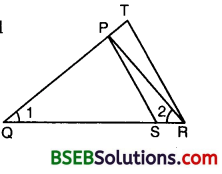Solution: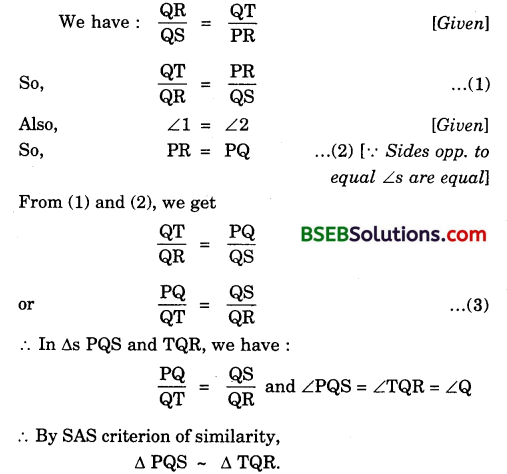Question 5.
S and T are points on sides PR and QR of ∆ PQR such that ∠P = ∠RTS. Show that ∆ RPQ ~ ∆ RTS.
Solution:
In ∆s RPQ and RTS, we have :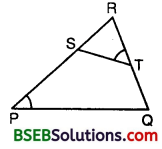∠RPQ = ∠RTS [Given]
∠PRQ = ∠TRS [Common]
∴ By AA criterion of similarity,
∆ RPQ ~ ∆ RTS.

Question 6.
In the figure, if ∆ ABE ≅ ∆ ACD, show that ∆ ADE ~ ∆ ABC.Solution:
It is given that ∆ ABE ≅ ∆ ACD
AB = AC [∵ Corresponding parts of congruent triangles are equal]
So, $$\frac { AB }{ AD }$$ = $$\frac { AC }{ AE }$$ = or $$\frac { AB }{ AC }$$ = $$\frac { AD }{ AE }$$
∴ In As ADE and ABC, we have :
$$\frac { AB }{ AC }$$ = $$\frac { AD }{ AE }$$ [ ∵of (1) ]
and ∠BAC = ∠DAE [common]
Thus, by SAS criterion of similarity,Question 7.
In the figure, altitudes AD and CE of ∆ ABC intersect each other at the point P.
Show that: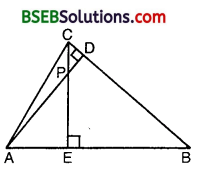(i) ∆AEP ~ ∆ CDP
(ii) ∆ ABD ~ ∆ CBE
(iii) ∆ AEP ~ ∆ ADB
(iv) ∆ PDC ~ ∆ BEC
Solution:
(i) In ∆s AEP and CDP, we have :
∠AEP = ∠CDP = 90° [∵ CE ⊥ AB and AD ⊥ BC]
∠APE = ∠CPD [Vert. opp. ∠s]
∴By AA criterion of similarity, we have :
∆ AEP ~ ∆ CDP.

(ii) In ∆s ∆BD and CBE, we have :
∠ABD = ∠CBE [Common angle]
∴By AA criterion of similarity, we have :
∆ ABD – ∆ CBE.

(iii) In ∆s AEP and ADB, we have :
∠AEP = ∠ADB = 90° [∵AD ⊥ BC and CE ⊥ AB]
∠PAE = ∠DAB [Common angle]
∴By AA criterion of similarity, we have :

(iv) In ∆s PDC and BEC, we have :
∠PDC = ∠BEC = 90° [∵ AD ⊥ BC and CE ⊥ AB]
∠PCD = ∠ECB [Common angle]
∴ By AA criterion of similarity, we have :
∆ PDC – ∆ BEC.

Question 8.
E is a point on the side ALD produced of a parallelogram ABCD and BE intersects CD at F. Show that ∆ ABE ~ ∆ CFB.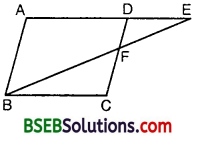Solution:
In ∆s ABE and CFB, we have:
∠AEB = ∠CBF [Alt. ∠s]
∠A = ∠C [Opp. ∠s of a gm]
∴By AA criterion of similarity, B we have :
∆ ABE – ∆ CFB.

Question 9.
In the figure, ABC and AMP are two right triangles, right angled at B and M respectively. Prove that :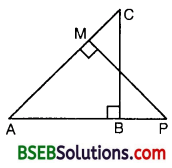(i) ∆ ABC ~ ∆ AMP
(ii) $$\frac { CA }{ PA }$$ = $$\frac { BC }{ MP }$$
Solution:
(i) In ∆s ABC and AMP, we have :
∠ABC = ∠AMP = 90° [Given]
and ∠BAC = ∠MAP [Common ∠s]
∴By AA criterion of similarity, we have :
∆ ABC – ∆ AMP.

(ii) We have :
∆ ABC ~ ∆ AMP [As proved above]
So, $$\frac { CA }{ PA }$$ = $$\frac { BC }{ MP }$$ [In similar triangles, correspondings sides are proportional.Question 10.
CD and GH are respectively the bisectors of ∠ACB and ∠EGF such that D and H lie on sides AB and FE of A ABC and ∆ EFG respectively. If ∆ ABC ~ ∆ FEG, show that :
(i) $$\frac { CD }{ GH }$$ = $$\frac { AC }{ FG }$$
(ii) ∆ DCB ~ ∆ HGE
(iii) ∆ DCA ~ ∆ HGF
Solution:We have : ∆ ABC ~ ∆ FEG
So, ∠A = ∠F
and ∠C = ∠G
∴$$\frac { 1 }{ 2 }$$ = $$\frac { 1 }{ 2 }$$ ∠G
or ∠1 = ∠3 and ∠2 = ∠4 … (2)
[∵ CD and GH are bisectors of ∠C and ∠G respectively]
∴ In As DCA and HGF, we have :
∠A = ∠F
∠2 = ∠4 [From (2)]
∴By AA criterion of similarity, we have :
∆ DCA – ∆ HGF
which proves the (iii) part.
We have : ∆ DCA ~ ∆ HGF [As proved above]
So, $$\frac { AC }{ FG }$$ = $$\frac { CD }{ GH }$$ or $$\frac { CD }{ GH }$$ = $$\frac { AC }{ FG }$$
which proves the (i) part.
In As DCB and HGE, we have :
∠1 = ∠3 [From (2)]
∠B = ∠E [∵ ∆ ABC ~ ∆ FEG]
∴By AA criterion of similarity, we have :
∆ DCB ~ ∆ HGE
which proves the (ii) part.

Question 11.
In the figure, E is a point on side CB produced of an isosceles triangle ABC with AB = AC. If AD ⊥ BC and EF ⊥ AC, prove that ∆ ABD ~ ∆ ECF.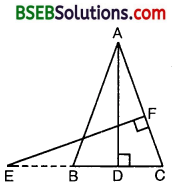Solution:
Here, ∆ ABC is isosceles with AB = AC.
∴ ∠B = ∠C
In ∆s ABD and ECF, we have :
∠ABD = ∠ECF [∵ ∠B = ∠C]
∠ADB = ∠EFC = 90° [∵ AD ⊥ BC and EF ⊥ AC]
∴ By AA criterion of similarity,
∆ ABD ~ ∆ ECF.

Question 12.
Sides AB and BC and median AD of a triangle ABC are respectively proportional to sides PQ and QR and median PM of ∆ PQR (see figure). Show that ∆ ABC ~ ∆ PQR.Solution:
Given : AD is the median of ∆ ABC and PM is the median of ∆ PQR such that
$$\frac { AB }{ PQ }$$ = $$\frac { BC }{ QR }$$ = $$\frac { AD }{ PM }$$
To prove : ∆ ABC ~ ∆ PQR∴ By SSS criterion of similarity, we have :
∆ ABD ~ ∆ PQM
So, ∠B = ∠Q [Similar ∆s have corresponding ∠s equal]
Also, [Given]
$$\frac { AB }{ PQ }$$ = $$\frac { BC }{ QR }$$ [Given]
∴ By SAS criterion of similarity, we have :
∆ ABC ~ ∆ PQR.Question 13.
D is a point on the side BC of a triangle ABC such that ∠ADC = ∠BAC. Show that CA² = CB.CD.
Solution:
In ∆s ABC and DAC, we have :
∠BAC = ∠ADC and ∠C = ∠C
∴ By AA criterion of similarity, we have :∆ ABC – ∆ DAC
So, $$\frac { AB }{ DA }$$ = $$\frac { BC }{ AC }$$ = $$\frac { AC }{ DC }$$
Now, from $$\frac { BC }{ AC }$$ = $$\frac { AC }{ DC }$$
$$\frac { CB }{ CA }$$ = $$\frac { CA }{ CD }$$
or CA² = CB x CD.

Question 14.
Sides AB and AC and median AD of a triangle ABC are respectively proportional to sides PQ and PR and median PM of another triangle PQR. Show that ∆ ABC ~ ∆ PQR.
Solution:
Produce AD to a point E such that AD = DE and PM to a point N such that PM = MN (see figure). Join CE and RN.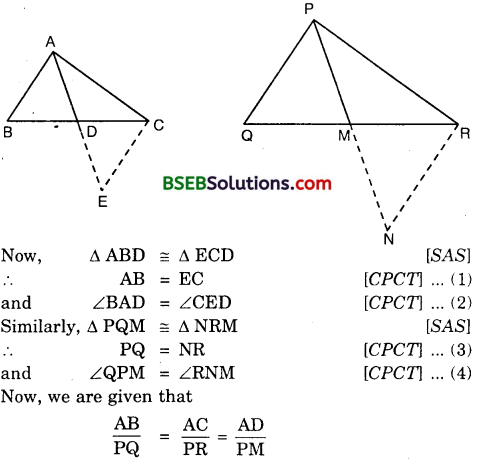So, from (1) and (3), we have :Question 15.
A vertical pole of length 6 m casts a shadow 4 m long on the ground and at the same time a tower casts a shadow 28 m long. Find the height of the tower.
Solution: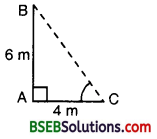Let AB be the vertical pole B and AC be its shadow. Also, let DE be the vertical tower and DF be its shadow. 6 m Join BC and EF. Let DE = x metres.
We have : AB = 6 m, AC = 4 m and DF = 28 m.
In As ABC and DEF, we have :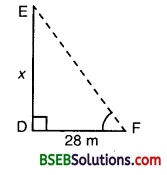∠A = ∠D = 90°,
and ∠C = ∠F
[∵ Each is the angular elevation of the sun]
∴ By AA criterion of similarity,
∆ ABC ~ ∆ DEF
or $$\frac { AB }{ DE }$$ = $$\frac { AC }{ DF }$$
So, $$\frac { 6 }{ x }$$ = $$\frac { 4 }{ 28 }$$ or $$\frac { 6 }{ x }$$ = $$\frac { 1 }{ 7 }$$
or x = 6 x 7 = 42.
Hence, the height of the tower is 42 metres.Question 16.
If AD and PM are medians of triangles ABC and PQR respectively, where ∆ ABC ~ ∆ PQR, prove $$\frac { AB }{ PQ }$$ = $$\frac { AD }{ PM }$$
Solution:
Given : AD and PM are the medians of As ABC and PQR respectively, where
∆ ABC ~ ∆ PQR
To Prove : $$\frac { AB }{ PQ }$$ = $$\frac { AD }{ PM }$$
Proof: In As ABD and PQM, we have :